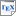[CDKM13b] T. Chen, M. Diciolla, M. Kwiatkowska and A. Mereacre. Verification of Linear Duration Properties over Continuous Time Markov Chains. Transactions on Computational Logic, 14(4), pages 1-35, ACM. Article 33. November 2013. [pdf] [bib] Downloads:pdf (602 KB)bib Links: [Google] [Google Scholar] [CiteSeer] Abstract. Stochastic modelling and algorithmic verification techniques have been proved useful in analysing and detecting unusual trends in performance and energy usage of systems such as power management controllers and wireless sensor devices. Many important properties are dependent on the cumulated time that the device spends in certain states, possibly intermittently. We study the problem of verifying continuous-time Markov chains (CTMCs) against linear duration properties (LDP), i.e. properties stated as conjunctions of linear constraints over the total duration of time spent in states that satisfy a given property. We identify two classes of LDP properties, eventuality duration properties (EDP) and invariance duration properties (IDP), respectively referring to the reachability of a set of goal states, within a time bound; and the continuous satisfaction of a duration property over an execution path. The central question that we address is how to compute the probability of the set of infinite timed paths of the CTMC that satisfy a given LDP. We present algorithms to approximate these probabilities up to a given precision, stating their complexity and error bounds. The algorithms mainly employ an adaptation of uniformisation and the computation of volumes of multi-dimensional integrals under systems of linear constraints, together with different mechanisms to bound the errors.